﻿ Geometrical Substantiation of <i>Phi</i>, the Golden Ratio and the Baroque of Nature, Architecture, Design and EngineeringInternational Journal of Arts

p-ISSN: 2168-4995    e-ISSN: 2168-5002

2011;  1(1): 1-22

doi:10.5923/j.arts.20110101.01### Geometrical Substantiation of Phi, the Golden Ratio and the Baroque of Nature, Architecture, Design and Engineering

Md. Akhtaruzzaman , Amir A. Shafie

Department of Mechatronics Engineering, Kulliyyah of Engineering, International Islamic University Malaysia, Kuala Lumpur, 53100, Malaysia

Correspondence to: Md. Akhtaruzzaman , Department of Mechatronics Engineering, Kulliyyah of Engineering, International Islamic University Malaysia, Kuala Lumpur, 53100, Malaysia.
 Email: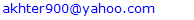Abstract

Golden Proportion or Golden Ratio is usually denoted by the Greek letter Phi (φ), in lower case, which represents an irrational number, 1.6180339887 approximately. Because of its unique and mystifying properties, many researchers and mathematicians have been studied about the Golden Ratio which is also known as Golden Section. Renaissance architects, artists and designers also studied on this interesting topic, documented and employed the Golden section proportions in eminent works of artefacts, sculptures, paintings and architectures. The Golden Proportion is considered as the most pleasing to human visual sensation and not limited to aesthetic beauty but also be found its existence in natural world through the body proportions of living beings, the growth patterns of many plants, insects and also in the model of enigmatic universe. The properties of Golden Section can be instituted in the pattern of mathematical series and geometrical patterns. This paper seeks to represent a panoptic view of the miraculous Golden Proportion and its relation with the nature, globe, universe, arts, design, mathematics and science. Geometrical substantiation of the equation of Phi, based on the classical geometric relations, is also explicated in this study. Golden Ratio and its chronicle, concept of Golden Mean and its relations with the geometry, various dynamic rectangles and their intimacy with Phi, Golden Ratio in the beauty of nature, Phi ratio in the design, architecture and engineering are also presented in this study in a panoptical manner.

Keywords: Golden Ratio, Golden Section, Golden Mean, Golden Spiral, Phi, Geometrical Validation of Phi, Fibonacci Number, Phi in Nature, Equation of Phi

Cite this paper: Md. Akhtaruzzaman , Amir A. Shafie , Geometrical Substantiation of Phi, the Golden Ratio and the Baroque of Nature, Architecture, Design and Engineering, International Journal of Arts, Vol. 1 No. 1, 2011, pp. 1-22. doi: 10.5923/j.arts.20110101.01.

### 1. Instauration

The interrelation between proportion and good looks has made a lot of discussion in science because of the accidental occurrence of the shapes in various designs of objects like books, paintings, edifices and so on. The designs are approximated by a rectangle shaped such that the ratio of its length and height is equal to the ‘Golden Ratio,’ φ = (1 + 51/2)/2 = 1. 6180339887 (approx.). The ‘φ’ also called as the divine proportion, golden section, golden cut, golden ratio, golden mean etc. which is the result of dividing a segment into two segments (A + B) such that A/B = (A+B)/A = 1. 6180339887 (approx.) where A > B .
The natural proportioning system provides the foundation of the work of many artists and designers. The proportion known as the Golden Mean has always existed in mathematics and in the physical universe and it has been of interest to mathematicians, physicists, philosophers, architects, artists and even musicians since antiquity. According to the great German mathematician Johannes Kepler (December 27, 1571 – November 15, 1630), geometry has two great treasures, theorem of Pythagoras and the division of a line into extreme and mean ratio . In the early days of the 19th century it was suggested that the Greek letter ‘φ’ (Phi), the initial letters of Phidias’s name, should be adopted to designate the golden ratio . In the medieval age and during the Renaissance, the ubiquity of ‘φ’ in mathematics aroused the involvement of many mathematicians. It is unknown exactly when the idea was first discovered and applied by mankind. It appears that the primitive Egyptian engineers may have used both Pi (π) and Phi (φ) in the structural design of the Great Pyramids. The Greeks based the design of the Parthenon (example of Doric architecture, the main temple of the goddess Athena built more than 400 years BC) on this proportion. Phidias, a Greek sculptor and mathematician (490-430 BC), studied Phi and applied this ratio in designing the sculptures of the Parthenon [2,4,5]. Plato (427-347 BC), in his views and understanding on natural science and cosmology presented in his Timaeus, one of his well-known dialogues, considered the golden section to be the most binding of all mathematical relationships and the key to the physics of the cosmos. In Elements, Euclid (325-265 BC) represented a line by dividing at the 0.6180399 point as the extreme and mean ratio. Afterwards the use of the term, Mean, appeared as Golden Mean to represent the ratio. He also proved the links of this number with the construction of a pentagram [2,6]. The Fibonacci Series was discovered, around 1200 AD, by Leonardo Fibonacci (1170-1250 AD) who was an Italian born mathematician. He found the interesting and mysterious properties of this numerical series latterly it is found that the series has a deep relation with Phi and the Golden Mean.
The concept of this ratio was first called as the Divine Proportion in the early 1500's when Da Vinci provided his illustrations for a dissertation which was published by Luca Pacioli in 1509 and entitled as De Divina Proportione. This book contains drawings of the five Platonic solids. It is predicted that Da Vinci was the first person who called it the Sectio Aurea, which is Latin terms indicating the Golden Section.
The Renaissance artists used the Golden Mean extensively in their paintings and sculptures to achieve balance and beauty. Leonardo Da Vinci used it to define all the fundamental proportions of his painting of The Last Supper, from the dimensions of the table, at which Christ and the disciples sat, to the proportions of the walls and windows in the background. Johannes Kepler (1571-1630 AD) who discover the elliptical nature of the orbits of the planets around the Sun also reflects the concept of the Divine Proportion. Martin Ohm (1792–1872 AD) who is believed to be the first man used the term ‘Goldener Schnitt’, Golden Section, to describe this proportion. The term Phi was not used before the 1900's until the American mathematician Mark Barr who used the Greek letter Phi to designate this proportion in his works. By this time the ubiquitous proportion was known as the Golden Mean, Golden Section and golden Ratio as well as the Divine Proportion. The proportion is said as Golden and Divine because of its unique properties to open the door of deeper understanding of beauty and spirituality of real world and universe.
Phi is the first letter of Phidias, who used the golden ratio in his sculptures. The Greek equivalent to the letter φ is F which is the first letter of the word Fibonacci. Phi is also the 21st letter of the Greek alphabet, and 21 is one of numbers in the Fibonacci series. Not only that, the summation of the digits of 21 is 3 (2 + 1 = 3) which is also one of the numbers of the Fibonacci series. ‘Phi’ also contains three alphabets. Again 21 holds the eighth position of the series and the number 8 is also a member of the Fibonacci sequence.
The characteristics of Phi have some interesting theological implications. The aesthetic primacy of the golden section was established empirically, and it was the very first topic of scientific psychological research as the new discipline emerged in the 19th century . Fechner was the first person who fixed his analytical gaze upon this task as early as the 1860's. Since that time, it has been the focus of a number of research fields such as Structuralism, Gestalt psychology, Behaviourism, Social psychology, Psychiatry and Neuroscience at various points in time . Phi continued to open new doors in the understanding of life, nature and the universe. It appeared in Roger Penrose's discovery of Penrose Tiles in the 1970's, which first allowed surfaces to be tiled in five-fold symmetry . It appeared again in 1984 in the aluminium-manganese alloy (Al6Mn), known as quasi-crystals, which was newly discovered form of matter . The relation between the Golden Ratio and the Black Holes is presented in 2011 by Nieto . This study represents the black hole solution in higher dimensions where a sparkling relation is found with the Phi. Anthropomorphic Robot research is one of the interesting fields where the humanoid robot sizing can be established based on Golden Proportion to make the social robot more presentable and acceptable to the general public [16,34,40,41]. Golden Ratio also used in particle swarm optimization . A recent study on ortho-cortex and para-cortex, two types of Cortex in Wool fibre, is conducted to have a better understanding on the relationship between the fibre structure and its properties where the fractal dimension of wool fibre shows the existence of Golden Mean in its structure . A theoretical foundation of applying a new control law, the Golden Section Adaptive Control (GSAC) law, is established in recent analysis to control a specific aircraft .
The next section, section 2, of this writing represents a succinct review on the relation between the Golden Ratio and the Geometry, concept of various dynamic rectangles and their links with the Phi ratio. Section 3 provides the substantiation of the equation of Phi contingent upon the classical geometrical approach. Phi as the baroque of the natural beauty and natural enigma is presented in section 4 with some representative patterns of the natural phenomena. In section 5, the uses and presence of the golden proportion in various artefacts, arts, portrait, design, architectural works and engineering are briefly introduced in a panoptic manner. Finally, the section 6 abridges the paper with some positive and opposite concept of the relation between the beauty and the Golden Proportion.

### 2. Phi and Its Relation with Geometry

#### 2.1. Concept of Golden Section

For a line segment, golden section can be considered as a point where the line is divided into two sections containing a unique property such as the ratio between the bigger segment and the shorter segment should be equal to the ratio between the line and its bigger segment [10-15,26,33-37]. The ratio is approximately 1.6180339887 and is indicated by the Greek letter φ. If a line AC is considered according to Figure 1, the point B is its golden section where the line is divided into two sections p and q (AB = p and BC = q). According to the theory, the ratio of p and q is equal to the ratio between (p + q) and p where p > q. This relation can be represented by the following equation,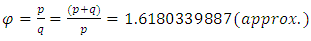(1)
From this relation it is clear that if any of the segments of the line AC is considered as 1, the other segment can easily be found with the value of φ. For example, if q is considered as 1 (q = 1) then p will be φ (p = φ) and if p is considered as 1 (p = 1) then the value of q will be the inverse of φ that is considered as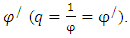From the most ancient time it is often been claimed that the golden section is the most aesthetically pleasing point at which the line is sectioned. Consequently, the idea has been incorporated into many art works, architectural design and mathematical analysis.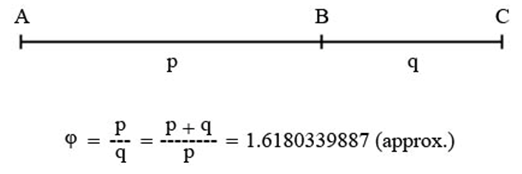Figure 1. A line segment AC is divided in the golden section point B

#### 2.2. Concept of Golden Rectangle and its Construction Strategy

A rectangle that is commonly associated with φ is the golden rectangle. It is a particular rectangle where the ratio between the length and width is exactly the same as the value of φ [13,14,15,37]. ABCD is a golden rectangle shown in Figure 2. The ratio between the two adjacent sides, AB and BC or CD and DA, is equal to the ratio between p and q or (p+q) and p. A straight line MN can be considered through the golden section of the ABCD that creates a square, ANMD, and a new smaller rectangle, MNBC, inside that golden rectangle. The side of the square is equal to the length of p and the new smaller rectangle having the adjacent sides equal to p and q (BC=MN=p and CM=BN=q) can be considered as the second level new smaller golden rectangle. Another straight line, IJ, also can be drawn between the two golden sections of the two sides AD and BC that will make another smallest square, MGJC, and another third level golden rectangle, GNBJ. This process can be continued for ever which will create smaller to smallest golden rectangles. The two lines MN and IJ intersect together at the point G and this intersection point is considered as the golden mean of the golden rectangle ABCD. Based on the different position of the golden section of a line, there can be at most four golden means in a golden rectangle. In Figure 2, the imaginary points G1, G2 and G3 are the other possible golden mean points of the golden rectangle, ABCD.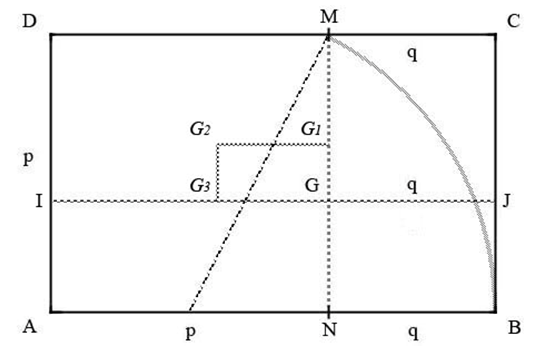Figure 2. Golden Rectangle, ABCD and the golden mean point G
There are some common techniques to draw a golden rectangle. Firstly, a perpendicular line having the length equal to the bigger segment of the base line divided at the golden section can be considered to draw the next larger golden rectangle. According to Figure 2 the base line AB is divided into p and q at the golden section point N. The perpendicular line, BC or AD, at any of the end point of the base line is equal to the length of p. So, the biggest possible golden rectangle, ABCD, can be drawn where the length of the rectangle is same as the base line. Secondly, one of the segments of the base line divided at the golden section can be folded at 90° angle with the other segment of the base line. Based on this L-shaped form, a golden rectangle can be drawn . In Figure 2 the bigger segment, AN, of the base line is folded at 90° angle with the smaller segment, NB. So, the L-shaped becomes as MNB and the new golden rectangle is MNBC. Thirdly, a square can be considered to draw a golden rectangle. Considering the midpoint of the base of the square as the centre of a circle arc and the length between the upper corner and the midpoint as the radius of that circle, an arc can be drawn that will intersect the extended base line of the square. Drawing a rectangle based on the new intersecting point and the square will make a golden rectangle [1,2,15]. In Figure 2, AN is considered as the base of the square MNAD, pM is the radius of the arc MB, where p is the centre. The arc MB intersects at the point B with the extended base line ANB. So, using the intersecting point the constructed rectangle becomes the golden rectangle, ABCD.
Golden rectangle is also known as whirling square rectangle because of the special property of subdividing into a reciprocal rectangle and a square [1,15]. The proportionally decreasing squares produce a spiral by using the arcs having radius as the length of the side of the squares [1,2]. Figure 3 shows the golden spiral AEGHIJKLO which is drawn inside the golden rectangle ABCD. BD and CF are the two diagonals of the main golden rectangle and the first smaller reciprocal golden rectangle, BCEF, respectively. The two diagonals intersect at the point O which is called the sink centre of the spiral and all other diagonals of the smaller golden rectangles must lie on these two diagonals. The ratio between the diagonal of the golden rectangle and its reciprocal is also in the same as the value of φ. In Figure 3 the largest arc, AE, of the spiral has the radius equal to the side of the big square AFED while the point F is the centre of that arc. The second biggest square, CGME, have the second arc of the golden spiral while the radius is equal to the side of that square and the point M is its centre. This process can be continued, and this will construct the spiral shape called the Golden Spiral.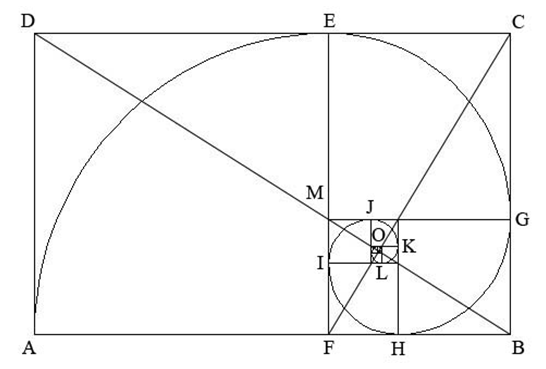Figure 3. Golden rectangle ABCD and golden spiral AEGHIJKLO

#### 2.3. Golden Triangles and Relations with Other Geometrical Patterns

Golden triangle is an isosceles triangle having 72° angle at the base and 36° angle at its zenith [1,15] or 36° angle at base and 108° angle at the apex. Figure 4 shows the two types of golden triangle ABC and XYZ. The ratio between any of its legs and its base is exactly same as the value of φ. Based on Figure 4 (a) and (b), Equation (1) can be written as,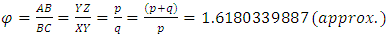(2)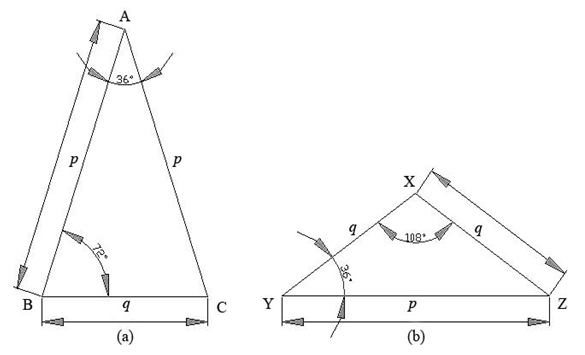Figure 4. Two different types of golden triangle (a) ABC and (b) XYZ
The value of φ is hidden in the component angles of the golden triangles. The absolute value of the cosine of any angle of the golden triangle is equal to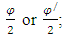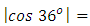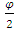and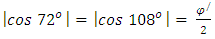. There are many examples in natural world where φ is intimately related with the shape of regular pentagon or pentagram. These pentagon and pentagram are possible to characterized using the golden triangle. The concept of golden triangle is very important because of the pentagonal symmetry in nature has often taken as an initial step to investigate the mystery of φ in the universe . The decagram and decagon also yield a series of golden triangles by connecting the centre point with any two adjacent edges [1,2,15].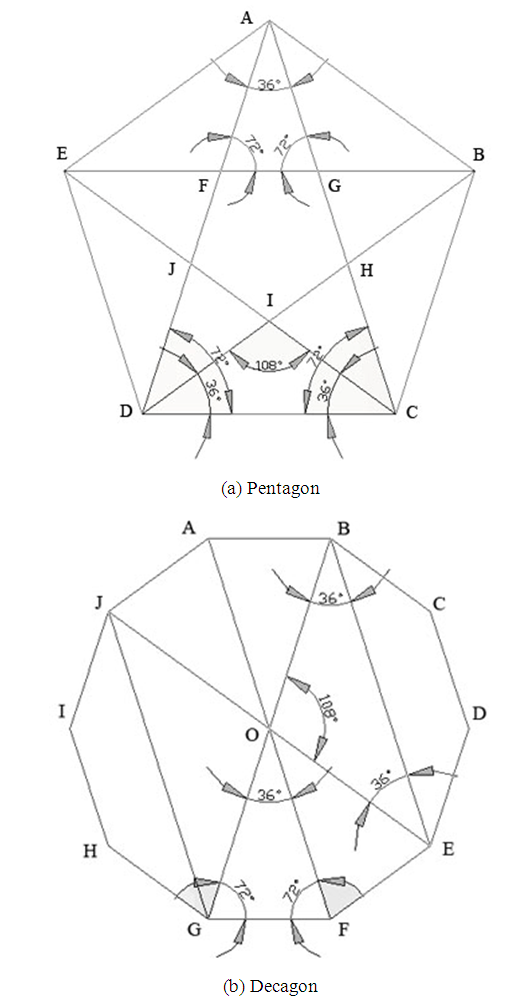Figure 5. A series of golden triangles construct the Regular Pentagon, Pentagram, Regular Decagon and Decagram
A golden triangle also can be considered as a whirling triangle where a spiral property is identified by subdividing into reciprocal triangles. A logarithmic spiral, also known as golden spiral, can be produced by joining the arcs having radius as the lengths of the sides of the reciprocal triangles [2,13]. Figure 6 (a) shows an isosceles triangle, ABC, having two base angles of 72 degrees. If the point D is a golden mean on the side AB; another reciprocal triangle can be drawn named BCD. A set of whirling triangles can be obtained by continuing this fashion which will produce the golden spiral ABCDEFO, shown in Figure 6 (b). The intersecting point, O, of the two lines CM and DN, is considered as the sink centre of the spiral where M and N are the midsection points of the sides AB and BC respectively. There could be only two sink centres of the two possible spirals for a golden triangle.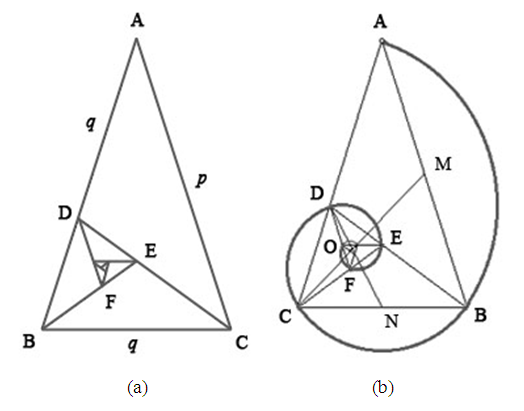Figure 6. Golden spiral formed on the whirling golden triangle

#### 2.4. Golden Angle and Golden Ellipse

A circle can be divided into two arcs in the proportion of the golden ratio, where the smaller arc marks a central angle of 137.5 degrees which is considered as golden angle. A Golden Section Ellipse is an ellipse drawn inside a Golden Section Rectangle where it has the same proportion of the major and minor axis as 1:1.618. A Golden Triangle is possible to draw inscribing in a Golden Ellipse that inscribes in a Golden Rectangle [2,13,15].

#### 2.5. Concept on Dynamic Rectangles

Based on the rational and irrational number of the proportion, a rectangle can be considered as either a static rectangle or a dynamic rectangle. Static rectangles have ratios of rational fractions such as 1/2, 2/3, 3/3, 3/4 etc. Dynamic rectangles are the rectangles with ratios of irrational fractions like √2, √3, √5, φ (golden section) etc. [1-3]. Static rectangles do not produce a series of visually pleasing ratios of surfaces while subdividing. On the other hand, dynamic rectangles produce an endless amount of visually pleasing harmonic subdivisions and surface ratios. There is a great intimacy between the √5 and φ both in mathematics and geometry. √2 rectangles possess an especial property, like Golden Rectangle, of being endlessly subdividing into proportionally smaller rectangles. It is possible to get two smaller √2 rectangles while dividing into half, dividing into forth resulting four smaller √2 rectangles and so on. The proportions of the √2 rectangle are approximately 1:1.42. √3, √4 and √5 rectangles also can be subdivided into three √3 rectangles, four √4 rectangles and five √5 rectangles respectively where the dividing process can be continued endlessly [2,3]. The proportions of the √3, √4 and √5 rectangles are approximately 1:1.732, 1:2 and 1:2.236 respectively.
Any of these rectangles can easily be drawn depending on its immediate antecedent such as √5 rectangles can be formed easily from √4 rectangles, √4 rectangles from √3 rectangles etc. Drawing a √2 rectangle depends on a square which also can be considered from √1 rectangle. Figure 7 shows the various dynamic rectangles and their construction strategies.
Beginning with a square having a diagonal BD, shown in Figure 7 (a), a √2 rectangle can be constructed. An arc DF having the radios BD touches at the point F on the baseline of the squire ABCD. A new rectangle with the point F can be formed as ABFE which is considered as a √2 rectangle. Similarly, another diagonal, BE, can be drawn that can be considered as the radios of the arc EG which is touching at the point G on the base line of the √2 rectangle. Now the new rectangle ABGH is obtained which is the √3 rectangle. The same procedure will construct the √4 and √5 rectangles as shown in Figure 7 (a).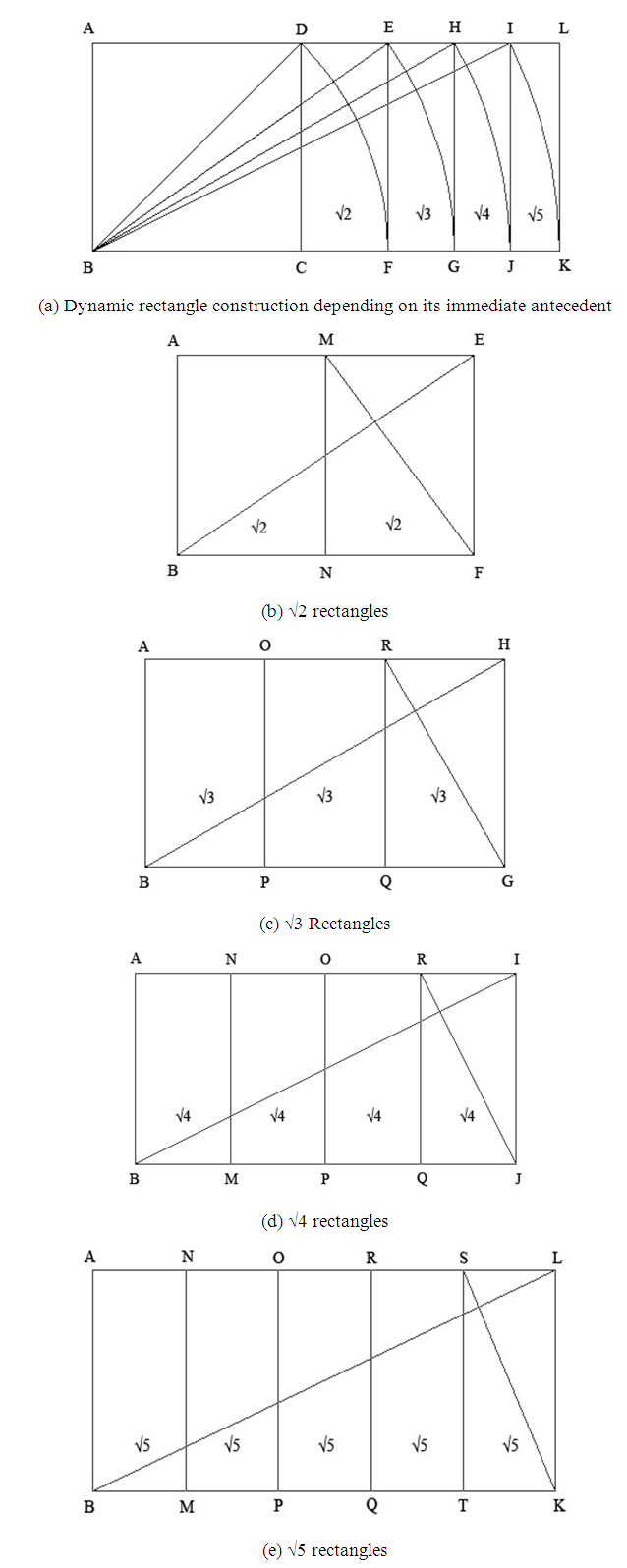Figure 7. Representation and construction of various dynamic rectangles

### 3. Geometrical Substantiation of the Equation of Phi

Phi is one of the great treasures of Geometry. As shown in Equation (3), the value of Phi has a close relation with √5 [1,28,29]. The same concept also can be applied to draw a golden rectangle which could be the geometrical proof of the equation of Phi.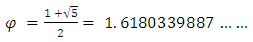(3)
If the two numbers 1 and √5 are added together and the result of the addition is divided by 2, the final result will become as the value of Phi. Now, if a square is considered as 1, a root 5 rectangle is considered as √5 and the golden rectangle is taken as the replacement of φ, the Equation (3) can be written as,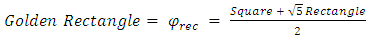(4)
Figure 8 (a) and 8 (b) show the square ABCD and the √5 rectangle CDEF respectively where the width of the √5 rectangle is equal to the side of the square, AB=CD=EF. By merging the square and the √5 rectangle it is possible to get another new rectangle called ABFE shown in Figure 8 (c). If the new rectangle is divided into two equal sections, the newly formed rectangles will be the Golden Rectangles. In Figure 8 (c), the GH line is acting as a divider of the merged rectangle ABFE and the resulting rectangles ABHG and GHFE becomes as two new Golden Rectangles.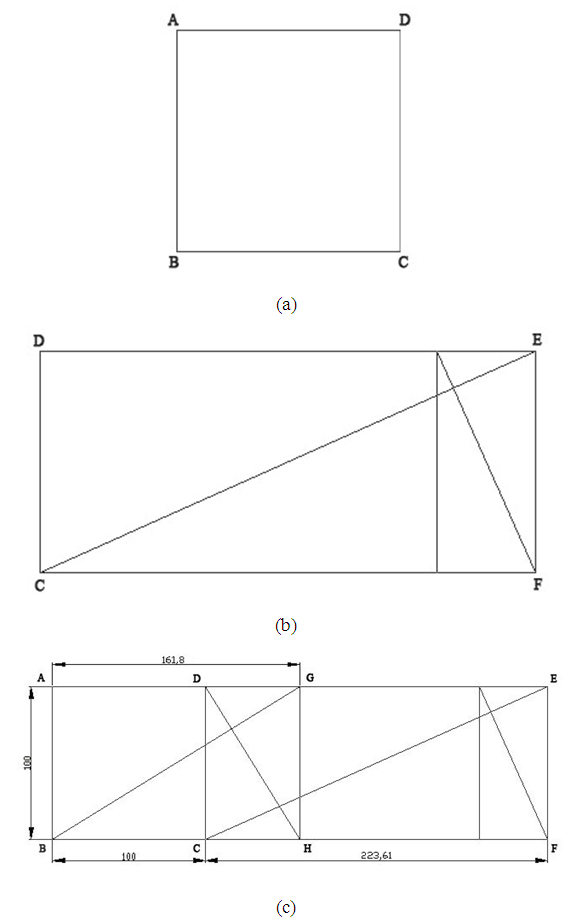Figure 8. (a) A square, (b) a Root 5 rectangle and (c) Golden Rectangle construction based on the equation of Phi
If the sides of a square are considered as ‘a’, then: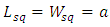(5)
Here Lsq and Wsq indicating the length and width of the square respectively. Starting from the square it is possible to obtain a √5 rectangle shown in Figure 7 (a). So, the length and the width of the √5 rectangle can be represented by the following equations,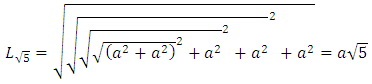(6)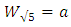(7)
So, the length of Golden Rectangle or Phi Rectangle (Lφ) will be the half of the summation of the length of √5 rectangle and the length of square. Here the width of the Phi Rectangle (Wφ) is equal to the width of the square as well as the width of the √5 rectangle.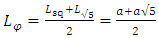(8)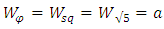(9)
Now from Equation (3), it can be written as,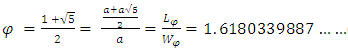(10)
Again, the length of the Golden Rectangle can be considered as ‘aφ’ where ‘a’ is the width of the rectangle. So, Equation (3) can be proofed from Equation (4),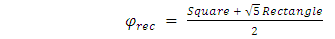(11)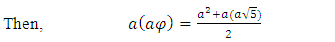(12)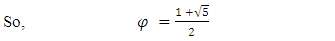(13)

### 4. Golden Section and the Beauty of Nature

In 12th century, the Leonardo Fibonacci questioned about the population growth of the rabbits under ideal circumstances, such as no predators to eat them or no dearth of food and water that would affect the growth rate. The answer of the question is the Fibonacci Sequence of Numbers, also known as Fibonacci Numbers, that starts from 1 and each new number of the series is simply the sum of the previous two numbers. So, the second number of the series is also 1, the sum of the previous 1 and 0 of the series. The sequence of the number looks like the series bellow.
0, 1, 1, 2, 3, 5, 8, 13, 21, 34, 55, 89, 144, 233, 377, 610, 987, 1597, 2584, ……………etc.
Fibonacci numbers are said as one of the Nature's numbering systems because of its existence not only in the population growth of rabbits, but also everywhere in Nature, from the leaf arrangements in plants to the structures in outer space. The special proportional properties of the golden section have a close relationship with the Fibonacci sequence. Any number of the series divided by the contiguous previous number approximates 1.618, near to the value of φ.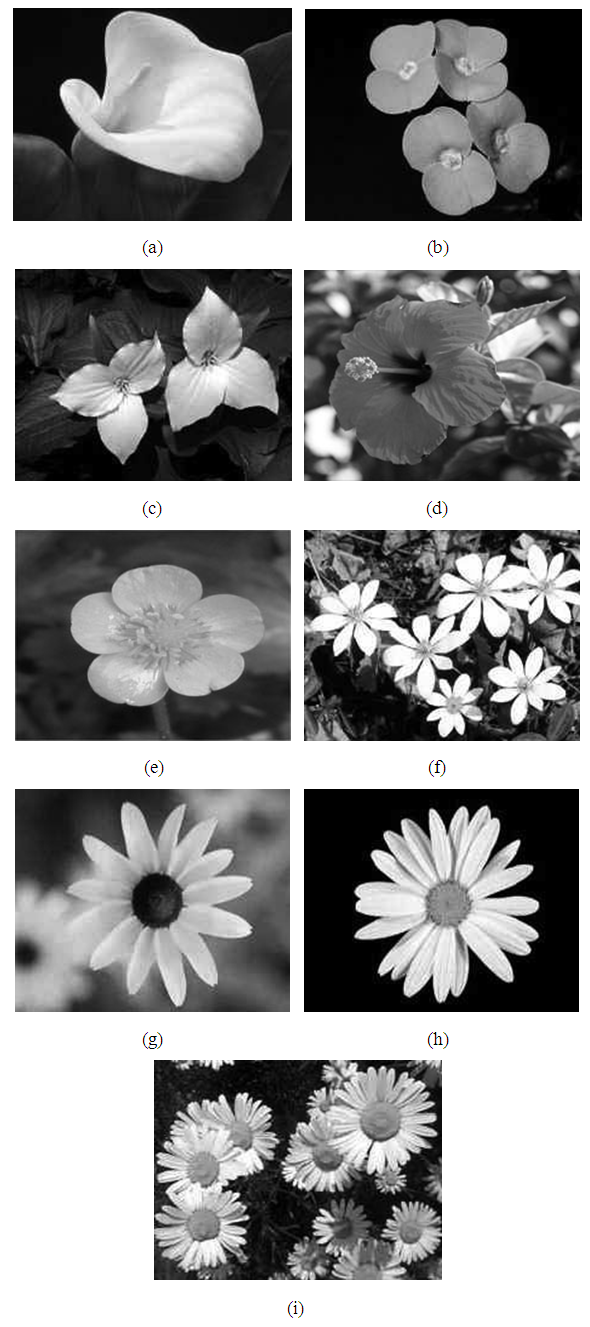Figure 9. Some flowers having different number of petals related to Fibonacci numbers, (a) White Calla Lily having one petal, (b) Euphorbia having two petals, (c) Trillium with three petals, (d) Hibiscus having five petals, (e) Buttercups with five petals, (f) Bloodroot with eight petals, (g) Black Eyed Susan having thirteen petals, (h) Shasta Daisy having 21 petals and (i) Daisy with 34 petals
Golden section preferences are considered as an important part of human beauty and aesthetics [20,23] as well as a part of the remarkable proportions of growth patterns in living things such as plants and animals [1-4,13,15,18]. Many flowers have the arrangement in petals that are to the Fibonacci numbers. Some display single or double petals. Three petals are more common like Lilies and Iris. Some have 5 petals such as Buttercups, Wild Rose, Larkspur and Columbines. Some have 8, 13, 21, 34, 55 and even 89 petals. All these numbers are consecutive Fibonacci numbers. The petal counts of Field Daisies are usually thirteen, twenty-one or thirty-four. The seed heads also follow the Fibonacci spiral arrangement. Other flowers having four or six petals also have a deep relation with Fibonacci numbers where they can be grouped into two and three respectively having two members each. Passionflower also known as Passiflora Incarnata is a perfect example having the existence of the Fibonacci Numbers. Figure 10 (a) shows the back view of Passionflower where the 3 sepals that protected the bud are at the outermost layer, then 5 outer green petals followed by an inner layer of 5 more paler green petals. The front view is shown in Figure 10 (b) where the two sets of 5 green petals are at outermost layer with an array of purple and white stamens, in the centre there are 5 greenish T-shaped stamens and at the uppermost layer has 3 deep brown carpels.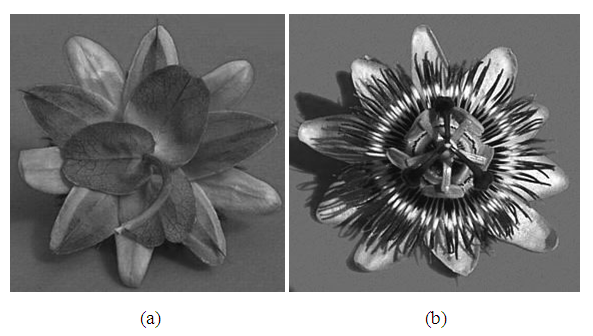Figure 10. Passionflower (Passiflora Incarnata) from the back (a) and front (b) having the examples of Fibonacci Numbers in nature
Romanesco Broccoli is one kind of vegetable that looks and tastes like a cross between broccoli and cauliflower. It is peaked in shape and has the arrangement with identical but smaller version of the whole thing that makes the spirals related to the Golden Spiral. The well grown Cauliflower has the shape almost as a pentagon which has an intimacy with the Golden Section and Golden Triangles. This enigma can easily be identified as shown in Figure 11. Fibonacci Numbers also can be found in some fruit’s seeds and their sectional arrangements. From the cross-sectional views of different fruits shown in Figure 12, Banana, Cantaloupe, Cucumber, Kiwano fruit also known as African cucumber, Watermelon have three sections. Some of the fruits have two subdivisions in each sectional part. Apple seeds are arranged like a pentagram shape that creates five sections. The pentagram structure also can be found in the Star Fruits. Okra also has five sections with the properties of a pentagon. Orange is divided into ten sections which can be grouped into five where each group contains two sub sections. Similarly, Okra’s seeds arrangement can be grouped into five as well.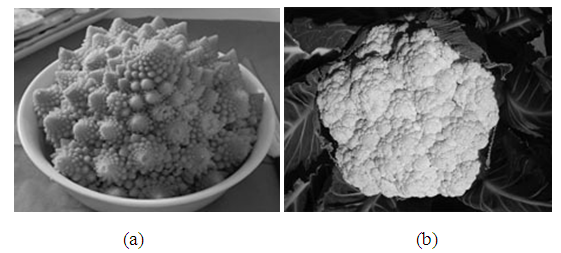Figure 11. (a) Romanesco Brocolli having the pattern of Golden Spiral, (b) Cauliflower having a shape of a pentagon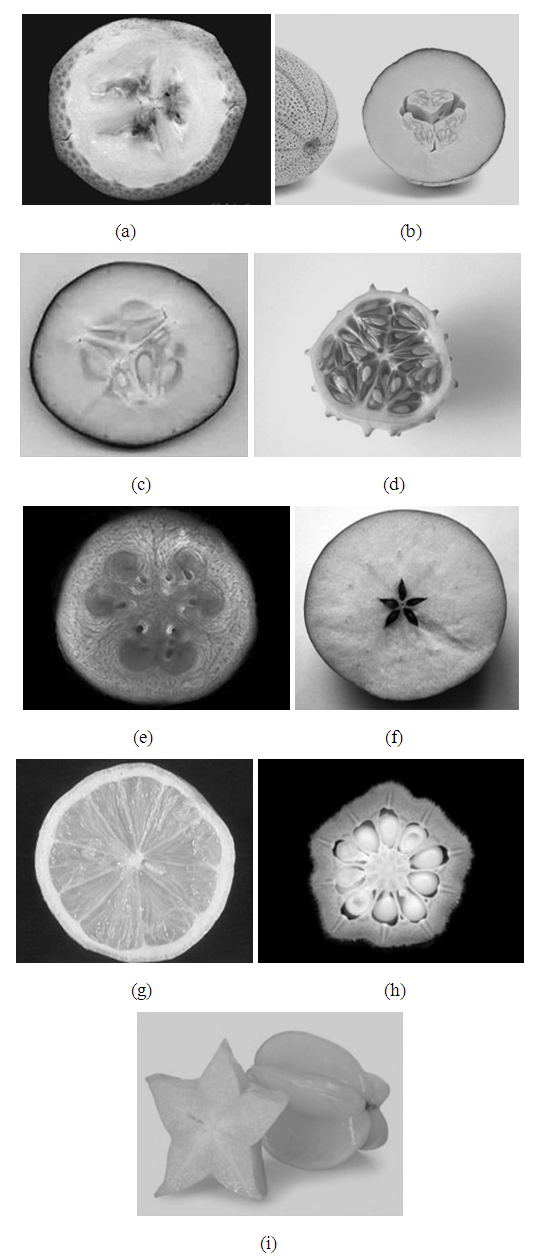Figure 12. Cross sectional view of some fruits and vegetables, (a) Banana has three sections, (b) Cantaloupe also have three sections, (c) Cucumber having three sections, (d) Kiwano fruit with three sections, (e) Watermelon also have three sections, (f) Apple having five seeds arranged as like a pentagram, (g) Orange having ten sections can be grouped into five, (h) Okra have the pentagon shape with ten seeds and (i) Star fruits have five sections with a pentagram shape
There is a direct correlation between the bi-directional spirals of the seed florets and Fibonacci Numbers. Not only that, the spiral has also a great relationship with the sequence of golden spiral. Figure 13 shows that the sunflower has 34 spirals in clockwise direction and 21 spirals in counter clockwise direction where these two numbers are the elements of the Fibonacci series.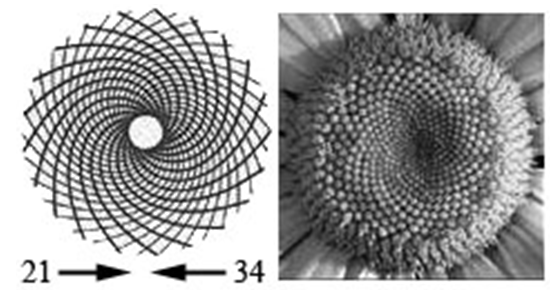Figure 13. Spiral arrangement of the seed florets of a Sunflower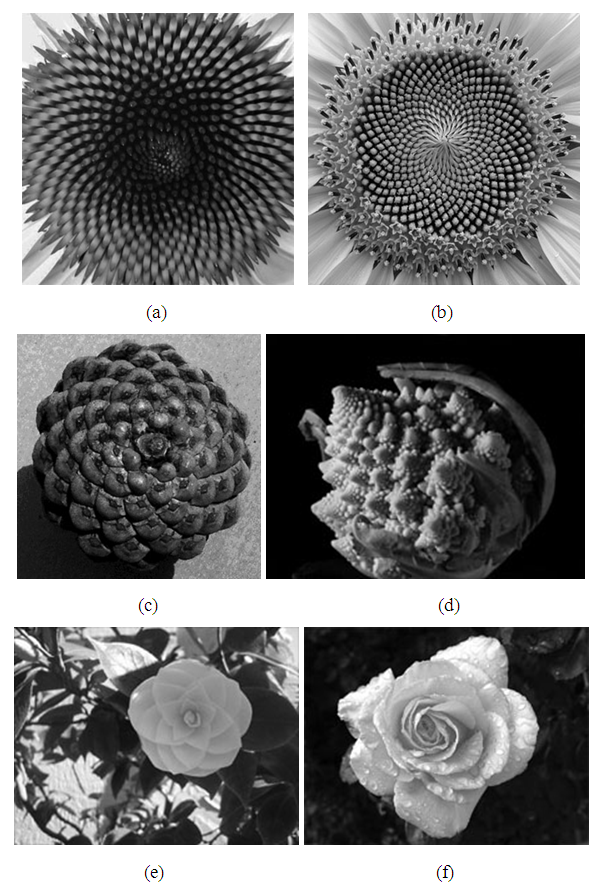Figure 14. Appearance of golden spiral in nature (a) Orange petals seed florets, (b) Sunflower seed florets, (c) Pine Cones, (d) Broccoli, (e) Golden Ratio flower and (f) Petals of a Rose 
The spiral happens naturally because each new cell is formed after a turn. Plants grow new cells in spirals format and this pattern is seen on the seed’s arrangement of the beautiful sunflower, orange petals seed florets, pine cones, broccoli and even in the petals of rose. Leaves, branches and petals also grow in spirals form too, so that the new leaves don't block the older leaves from the sun ray or the maximum amount of rain or dew gets directed down to the roots. If a plant has spirals, the rotation tends to be a fraction made with two successive Fibonacci Numbers, for example, 1-2, 3-5 or even 5-8 also common that getting close to the golden ratio.
Plants have distinct characteristics of Golden Ratio where they establish a Fibonacci sequence in the number of leaves. Even the eyes of a pineapple follow the golden ratio and golden spiral. Much of the things that are viewed as beautiful by the naked eye establish the factor that possesses the Golden Ratio in one way or another. The term phyllotaxis means "leaf arrangement" in Greek and was coined in 1754 by Charles Bonnet, a Swiss naturalist . In the 1830s, a scientist brothers found that each new leaf on a plant stem is positioned at a certain angle to the previous one and this angle is constant between leaves usually about 137.5 degrees. In the top view of the plant, shown in Figure 15, the angle formed between a line drawn from the stem to the leaf and a corresponding line for the next leaf, is generally a fixed angle which is known as the Divergence Angle or Golden Angle [3,15,18]. Figure 16, (a) to (d), show some succulent plants where this characteristic is clearly visible.Figure 15. Loosely distributed leaf’s structure where the successive leaves in the spiral are numbered and lined from the centre of the spiral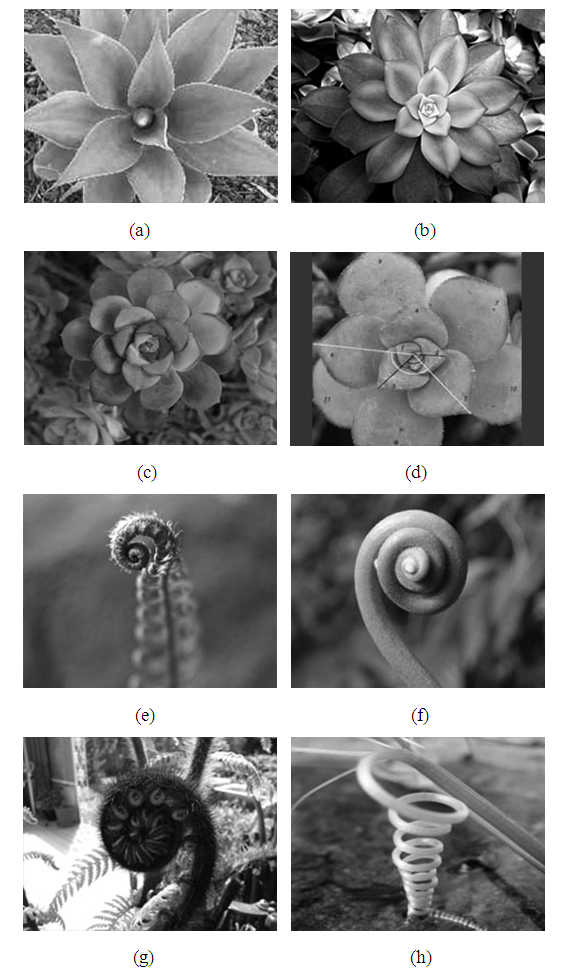Figure 16. Golden Angle and Golden Spiral are also found in some plants and ferns. (a), (b), (c) and (d) show various succulent plants having Golden Spirals and Angles in their leaf arrangement. (e), (f) and (g) show some Fern’s top. (h) Vine Tendrils [18,50]
Research has shown that buds placed along a spiral and separated by an angle of 137.5 degrees are packed most efficiently along the spiral. In 1868, Wilhelm Hofmeister suggested that new primordia, cells that will later develop into leaves or petals, always form in the least crowded spot along the growing tip of a plant called a meristem. This is also known as Hofmeister's rule . Because the plant is continuously growing, each successive primordium forms at one point along the meristem and then moves radially outward at a rate proportional to the stem's growth. Hofmeister's rule tells that the second primordium is placed as far as possible from the first, and the third is placed at a distance farthest from both the first and the second primordia. As the number of primordia increases, the divergence angle eventually converges to a constant value of 137.5 degrees and thus creates Fibonacci spirals . Various Fern tops and Vine Tendrils show almost the same characteristics of the Fibonacci spiral or Golden spiral. Not only that, Fibonacci spiral also found on some cactuses and some fruits. Most pineapples have five, eight, thirteen, or twenty-one spirals of increasing steepness on their surface. All of these are Fibonacci numbers . Figure 17 shows various types of Cactuses having almost the same properties of Golden spiral in their growth patterns.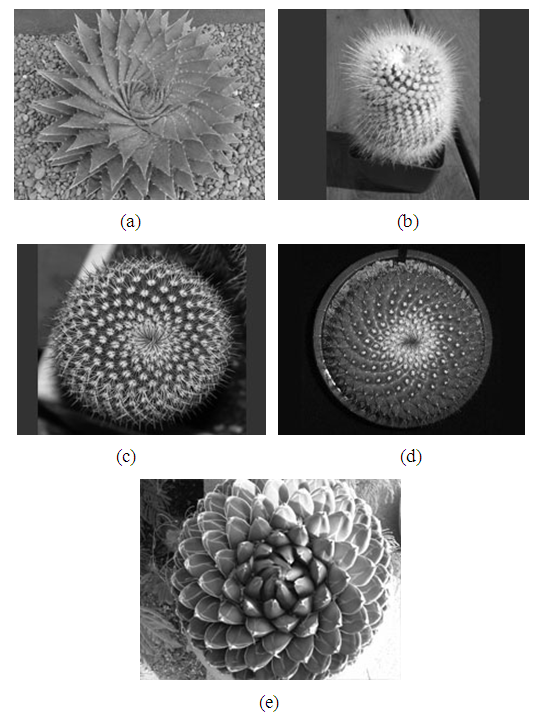Figure 17. Some various Cactuses are showing the existence of the Golden Spiral in their various growth patterns [18,50]
The Fibonacci numbers form the best whole number approximations to the Golden Section. Plants illustrate the Fibonacci sequence in the numbers and arrangements of petals, leaves, sections and seeds. Plants that are formed in spirals, such as pinecones, pineapples and sunflowers, illustrate Fibonacci numbers. Many plants produce new branches in quantities that are based on Fibonacci numbers. German psychologist Adolf Zeising, whose main interests were mathematics and philosophy, found the Golden Section properties in the arrangement of branches along the stems of plants and of veins in leaves . Figure 18 shows the existence of Golden Spiral and Golden Angle on the leaves of trees. Zeising also concentrated on the skeletons of animals and the branching of their veins and nerves, on the proportions of chemical compounds and the geometry of crystals, even on the use of proportion in artistic endeavours. He found that the Golden Ratio plays a universal and important role in all of these phenomena .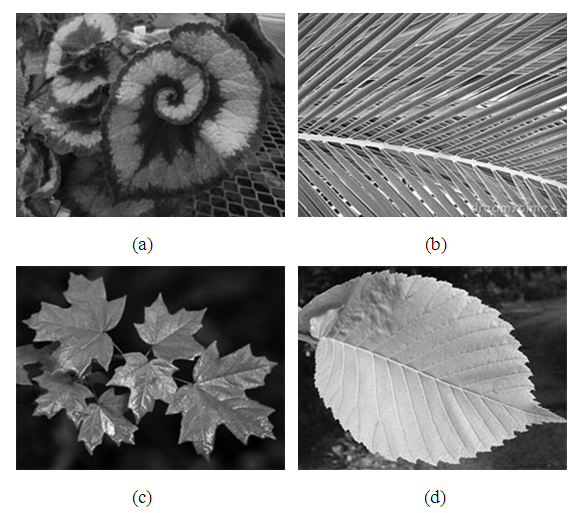Figure 18. (a) Spiral leaves of orchid, (b) Leaves of a Coconut tree having Golden angles, (c) Maple leaves with Golden angles between primary and secondary veins and (d) Golden Elm Leaf with Golden angles in veins arrangements
There are a lot of examples of the Golden Section or Divine Proportion found on animals, fishes, birds, insects, and even on some snails. The eye, fins and tail of a dolphin fall at Golden Sections of the length of its body. A penguin body also can be described by the Golden Ratio properties. The Rainbow Trout fish, shown in Figure 19 (h), also shows the same properties where three golden rectangles together can be fitted on its body where the eye and the tail fin falls in the reciprocal golden rectangles and square . The individual fins also have the golden section properties. An experiment on Blue Angle fish shows that the entire body of the fish fits perfectly into a golden section rectangle, shown in Figure 19 (g). The mouth and gill of the Angle fish are on the reciprocal golden section point of its body height .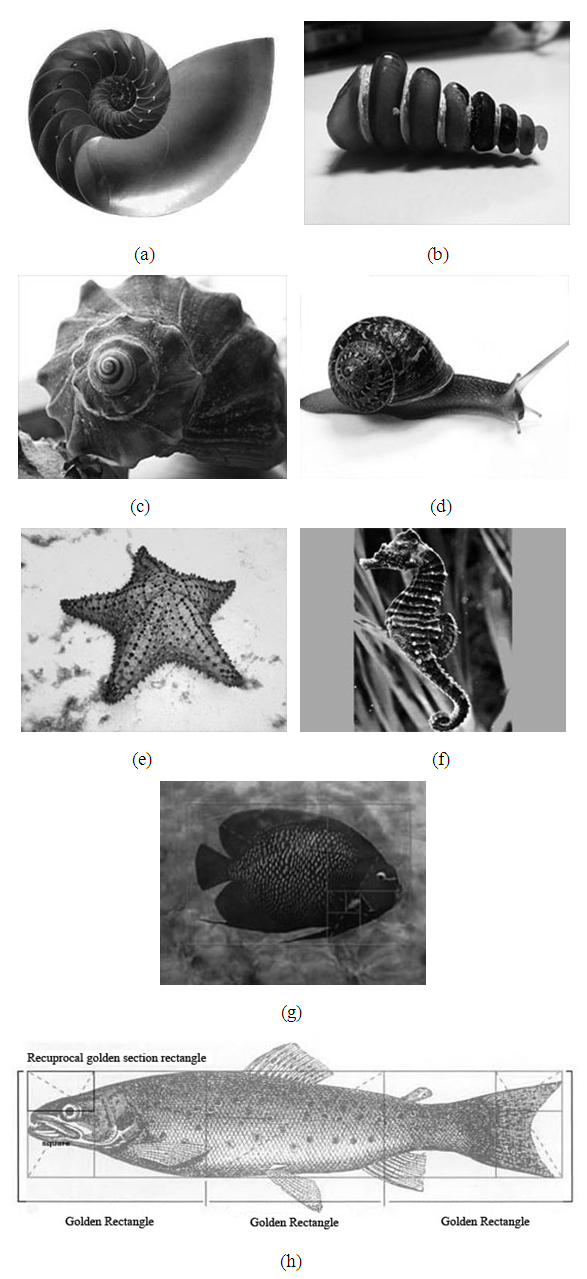Figure 19. (a), (b), (c) and (d) some natural shell and a garden snail having Golden Spiral like structure, (e) Star fish, (f) Sea Horse, (g) Blue Angle Fish having perfect Golden Section example and (h) Trout fish 
The growth patterns of natural shells are showing the logarithmic spirals of golden section properties and it is known as the theory of a perfect growth pattern . In his book, “The Curves of Life”, Theodore Andreas Cook describes the growth pattern as; “the essential processes of life….” . Shells like Chambered Nautilus, Conch Shell, Moon Snail Shell, Atlantic Sundial Shell show the spiral growth pattern where the first three have almost like the golden spiral form, shown in Figure 19 (a) (c) and (d). Tibia Shell spiral growth is not like golden spiral but the sections of the spiral body can be described by the golden mean properties . Golden spiral also be found on the tail of Sea Horses. The Star fish has the structure like a pentagram which has a close intimacy with golden ratio. The body sections of ants are to the Golden Ratio. The same properties also are found on the beautiful design of butterfly wings and shapes. The natural design of Peacock’s feather also goes to the golden ratio .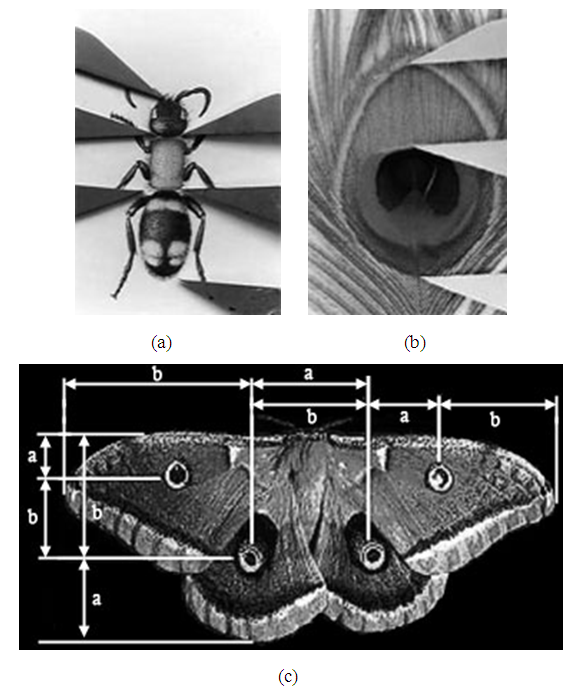Figure 20. Golden Section appears in (a) ant’s body parts, (b) peacock’s feather and (c) natural design of a butterfly [17,47,48]
The Golden Ratio is also found all throughout the physical universe. Weather patterns, Whirlpool have almost the same form as the golden spiral. Even the Sea Wave sometimes shows almost the same spiral pattern. The three rings of Saturn are designed naturally based on the Golden Ratio. The Galaxy, Milky Way, also has the spiral pattern almost like golden spiral. Relative planetary distances of Solar System also have the golden ratio properties.
The orbital distances of planets are generally measured from the Earth. Mercury is the first planet of the solar system where the Earth is on third. So, to take the measurements from Earth would be like starting the Fibonacci numbers from somewhere in the middle of the sequence. If the measurement is started from the first planet of the solar system, Mercury, a very special and interesting property will be outspread. The asteroid belt is a part of the solar system and the largest asteroid is Ceres, which is one third of the total mass of all the asteroids. So, Ceres could be the logical orbit. If the average of the mean planet orbital distances of each successive planet is taken in a relation to the one before it, the value will be following the golden ratio number. Mean distance in million kilometres as per NASA, Mercury 57.91, Venus 108.21, Earth 149.60, Mars 227.92, Ceres 413.79, Jupiter 778.57, Saturn 1,433.53, Uranus 2,872.46, Neptune 4,495.06, and Pluto 5,869.66. Relative mean distances where Mercury is considered at the beginning, 1.00, 1.86859, 1.38250, 1.52353, 1.81552, 1.88154, 1.84123, 2.00377, 1.56488, and 1.30580 respectively. Total relative mean distance is 16.18736 and the average is 1.61874 which is closer to the Golden ratio, 1.61803.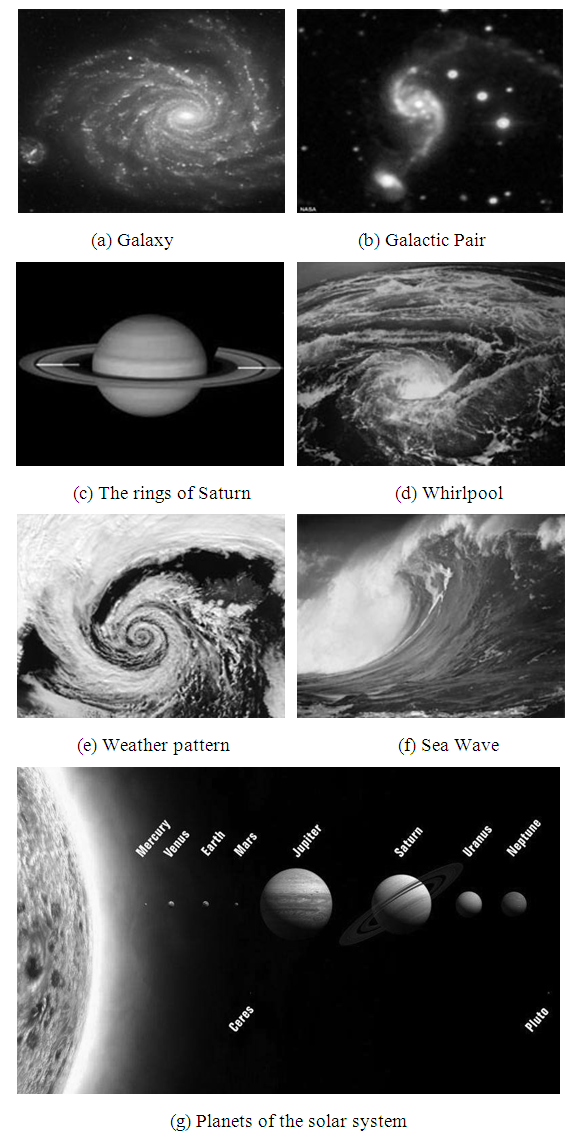Figure 21. Some example of the physical universe where Golden Section properties are exists [47,48]
The Fibonacci sequence can be considered more or less the beauty of nature. The human body also exemplifies the mesmerizing occurrence of Golden Ratio. Total height of the body and the distance from head to the fingertips make the same ratio as Phi. Again, the distances from head to naval and naval to hill also express the golden proportion [1,2,4]. The bones of fingers in human hand are related to each other by a ratio of Phi. For instance, the proportion between the forearm and upper arm also follows the rule of golden ratio and the same ratio appears between the hand and forearm. Human faces are also comprised of this ratio within the relationships between the eyes, ears, mouth and nose [17,22]. The human being is the most beautiful and most perfect instauration of Allah. Allah said in the Holly Quran;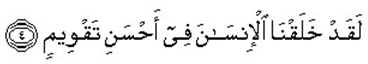[Surah At-Tin (95), Verse 8]. Meaning: We have indeed created human in the best of modules.
It is known that the Arabic text direction is from right to left. There are exactly 26 characters in this sentence and the word “Insana”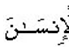means ‘man’ or ‘human’. This verse also has the Golden proportion where the word “Insana” comes at the golden mean of the sentence. The 26 characters can be divided into two sections p and q. The left section p has 16 characters started from the word “Insana” and q has 10 characters from the beginning to that word. The ratio between p and q is 1.6 which is closer to the golden number 1.618... Indeed, Quran is a miracle for its eloquence in language, for applicability and relevance of its verses in all spheres of human life, for its inimitable verses and in many other aspects. It does not need to prove as scientific, rather we need to guide by it.
The relationship of golden section is observed on the design of the human body shape and structure. It consists of one trunk, one head, one heart etc. Many parts of the body are in pair like arms, legs, eyes, buds. Legs and arms consist of three parts. Human fingers consist of three phalanxes, main, mean and nail. The length of all phalanxes of each finger relates to each other according to the rule of golden proportion. There are five appendages to the torso, the arms, legs and a head; five appendages on each of these, five fingers on hands, foot and five openings on the face. There exist five senses as sight, sound, touch, taste and smell. Five is a number of the Fibonacci series and the golden section is also computed using five, as shown in Equation (3). Many researchers of the human body mathematical regularities inscribed man into a pentagram. Such model was reflected in Leonardo da Vince and Durer's constructions.
Again, hand consists of three main parts: wrists, metacarpus and fingers. Human arm together with fingers consists of eight parts. Eight bones enter to the wrist structure which is connected with five metacarpal bones forming the basis of the palm. Five fingers are connected to metacarpal bones. Human has 12 pairs of ribs and sometimes it is claimed (without any strong evidence) that in the past the man had 13 pairs of ribs. Facial, middle ears and throat consists of total 21 bones as 14, 6 and 1 respectively. Human backbone with the skull consists of 34 bones, 8 Crania (skull), 24 Vertebrae, one Sacrum and one Coccyx. The total number of 55 (21 + 34 = 55) bones compound together to build up the base column of human body structure. All of these numbers 1, 2, 3, 5, 8, 13, 21, 34 and 55 are the numbers of the Fibonacci series. Basically, it is necessary to do research on the roots of the natural laws deeper and deeper to establish the relation with the golden proportion.
Dr. Stephen Marquardt, an eminent Oral surgeon in California, discovered that the height of the central incisor is in the Golden Proportion to the width of the two central incisors . This revelation has offered solutions to a host of dental aesthetic problems. The Golden Proportion grids show the inter-tooth relationship between the eight teeth of the anterior aesthetic segment whereas the rectangle confirms the width of the incisors, related to their height . The combination of grid and rectangle will mutually confirm the aesthetic solution with a certainty that it will look attractive and natural. These grids explained in the paper “Dental aesthetics and the golden proportion” are complementary to the Golden Proportion rectangle. The combination of the two gives a powerful tool to confidently determine good aesthetics. The four front teeth, from central incisor to premolar are the most significant part of the smile and they are in the Golden Proportion to each other. Dr Mc Arthur 1985 wrote an article "Maxillary and Mandibular Teeth Widths" in which he explained that the average ratio of upper central incisor to lower central incisor is 1.62. Shoemaker in 1987 wrote a series of articles promoting the use of the Golden Proportion as an adjunct to cosmetic Dentistry. Amoric (1989) in his article, "Le nombre d'or", showed many Golden Proportions in cephalometric tracings at various stages of facial growth and also included geometrical propositions.
The Annals of Plastic Surgery 1989 include a fascinating study by Kawakami et al. who investigated the Golden Proportion balance between eyes, nose and mouth in the facial appearance of typical Japanese individuals and comparing the ratios to Caucasians, each ratio was then used for pre and post-operative aesthetic analysis using Moire' topography. Dr Jack Preston, in 1993, was influenced by the studies of Stephen Marquardt who developed a Golden Proportion Pentagon Grid for Facial plastic surgery. Dr Yosh Jefferson, in 1996, illustrates an article in the Journal of General Orthodontics with many Golden Proportion diagrams and computer generated photographs giving an ideal picture of the whole head. His work was also supported with cephalometric tracings.
It was also found that the human heart beats are in the Golden Proportion rhythm. Figure 22 (j), (k) and (l) show the Golden Proportion gauge on Electrocardiogram (ECG) tracings of a normal heart. The DNA cross-section is also based on Phi ratio. A decagon is possible to draw from a cross-sectional view from the top of the DNA double helix. A decagon is in essence two pentagons, with one rotated by 36 degrees from the other. So, each spiral of the double helix must trace out the shape of a pentagon. The DNA molecule is also based on the Golden section. It measures 34 angstroms long and 21 angstroms wide for each full cycle of its double helix spiral model where 34 and 21 are the numbers in the Fibonacci series and their ratio is 1.6190476, closer to the ratio of Phi, 1.6180339….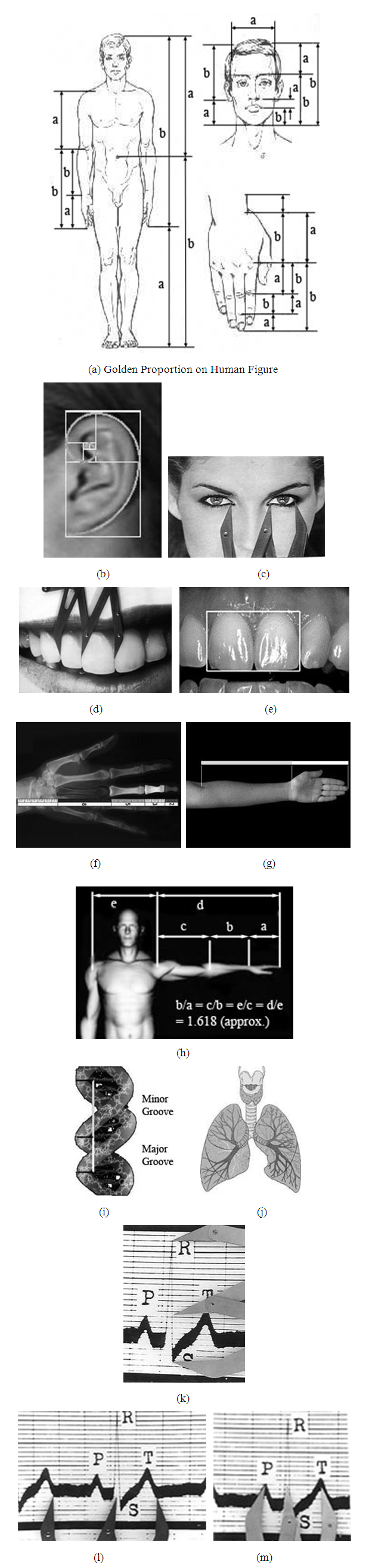Figure 22. Golden Section in human body parts (a) Human body, face and fingers, (b) Spiral on human ear, (c) Eye position on human face, (d) and (e) Golden ratio on human teeth, (f) X-ray image of human fingers, (g) and (h) Human hand and shoulder, (i) DNA spiral, (j) Human lung, (k), (l) and (m) Electrocardiogram (ECG or EKG) tracings [47,48,51]
The position of Makkah (Mecca) on our Globe also exemplifies the miracle of Golden ratio. According to the geography, the Equator is a line around the earth, at an equal distance from both poles, is the latitude line given the value of 0 degree. There are 90 degrees of latitude going to the north and south from the Equator. So, the total degrees between north and south poles are 180. Similarly, the Earth is divided equally into 360 degrees of longitude and there are 180 degrees of longitude to the east and west from the Prime Meridian. The golden mean of the earth is possible to calculate based on the following equations;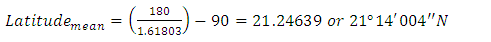(11)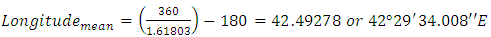(12)
This Golden Ratio Point of the World lies on Makkah according to the map of latitude and longitude which is the common determinant of mankind for location. The Phi Matrix program, used for displaying golden ratio of pictures and drawings, also shows that the Golden Ratio Point of the longitude and latitude map of the World is on the Makkah. Figure 23 (d) shows the Google Earth image where the large arrow indicating the Golden Mean point of the Globe.
Again, according to the Google Earth, the latitude and longitude position of the Holly Kaaba are lat: 21.42251 and lon: 39.8262 or 21o25’21.04”N and 39o49’34.32”E. The position of the Makkah city centre, pointed on Google Earth, is lat: 21.42737 and lon: 39.81483 or 21o25’38.55”N and 39o48’53.41”E. For both of these positions, the ratio between the south and the north parts is almost 1.624 or 1.6 (approx.) and the ratio between the distance to the west and the east is approximately 1.568 or 1.6 (approx). Both of these ratios are closer to the value of the Golden Ratio, 1.61803. The distance between the Golden Mean point of the Earth and the Holly Kaaba is just a few kilometres, 277km, and if a straight line is drawn between these two points, shown in Figure 23 (d), Mena falls just on it.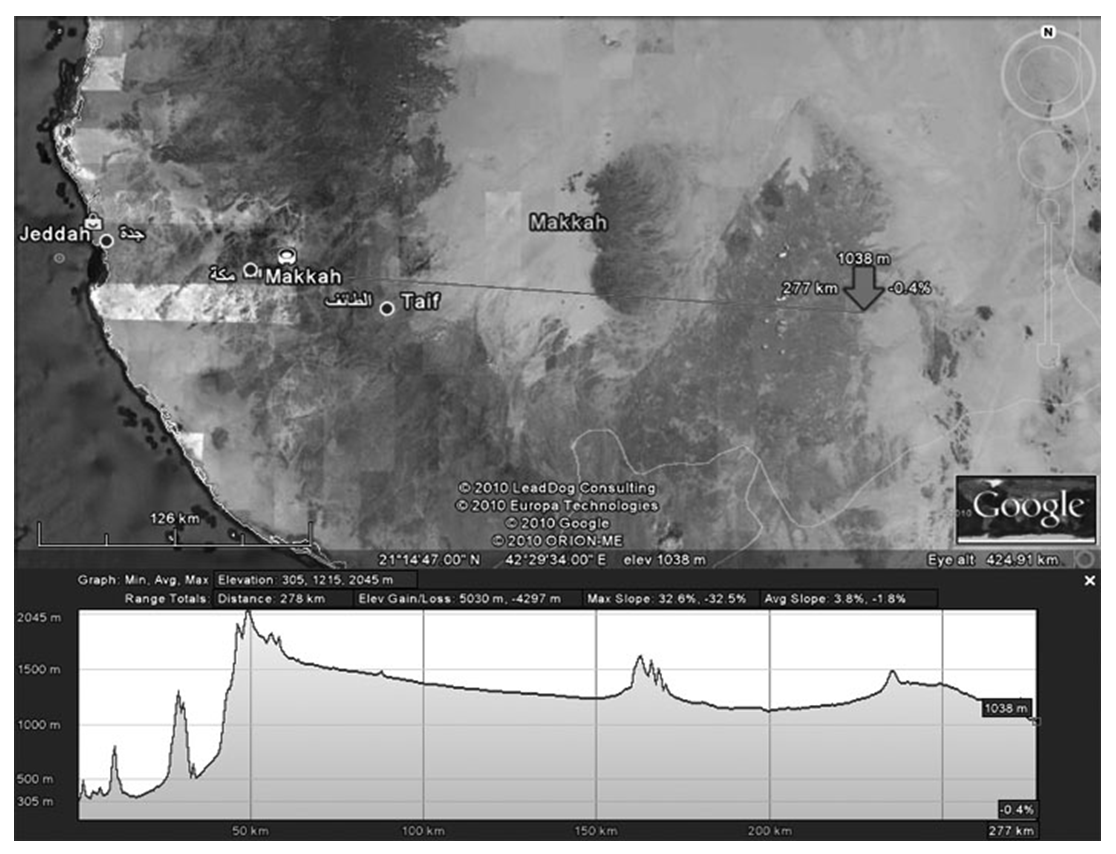Figure 23. Google Earth image indicating the latitude and longitude of Mecca and Golden Mean point of the Globe
The relation between the City of Mecca and the Golden Ratio is clearly stamped in the Surah Al-Imran (3), verse 96. Allah says;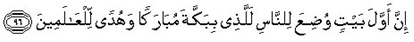Meaning is, “Lo! The first Sanctuary appointed for mankind was that at Mecca, a blessed place, guidance to the people.” The total number of all letters of this verse is 47. Calculating the golden ratio of total letters, it is clear that the word of Mecca is implied at (47 / 1.618) = 29. There are 29 letters from the beginning of the verse till the word,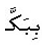which indicates the Mecca and contains three characters itself.

### 5. Golden Ratio in Design, Architecture and Engineering

If the relative ratio is 1.618 for the components of any structure, the form will be convenient to Golden Ratio, says as the perfect design. One of the 5 platonic solids, dodecahedron, is described by Plato in the "The Phaedo" (110 B.) where he refers to a ball having twelve pentagonal faces. This was the precursor to the design of the modern football, made with a various number of pentagons as shown in Figure 24 (b). Golden ratio is also used in the design of violins, guitars and even in the design of high quality speaker wire.
Le Corbusier, one of the famous architects, also represented the divine proportion onto his design, Chaise Longue in 1929, shown in Figure 24 (h). The proportion of the chaise relates to the harmonic subdivision of a golden rectangle where the width of the rectangle becomes the diameter of the arc that is the frame of the chaise . Plywood Chair, designed by Charles Eames in 1946, also reflects the application of the golden ratio in its design strategies . The chair back fits perfectly into a golden section rectangle.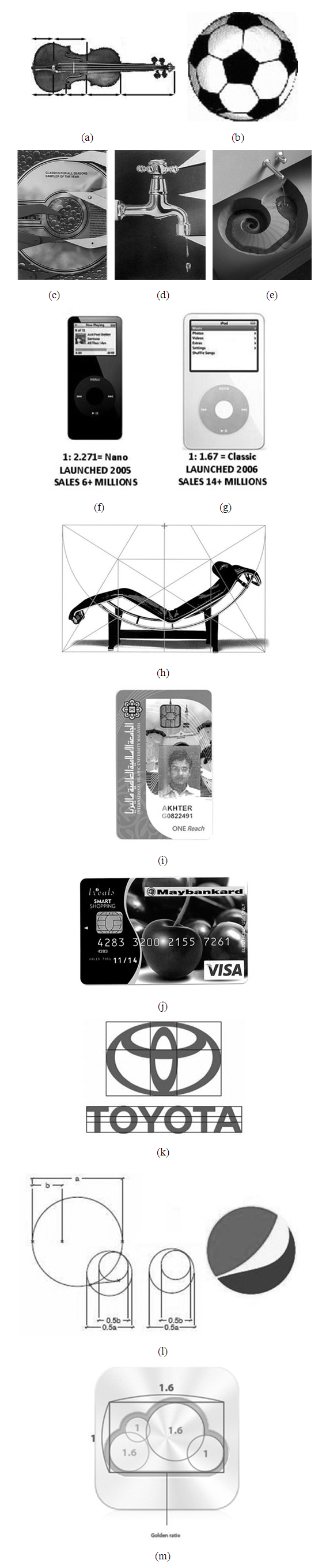Figure 24. (a) Golden Ratio on a Violin, (b) Pentagon appears on Football, (c) Golden Ratio on Compact Disk (CD) design, (d) Water tap, (e) Spiral design of a kitchen sink, (f) and (g) two different design of APPLE iPod, (h) Chaise Longue design based on Golden Ratio, (i) and (j) ID Card and Visa Debit Card, (k) and (l) Toyota and PEPSI logo design based on Golden Ratio, (m) Legendary design of Apple iCloud logo [1,47,48]
Credit cards are also in the shape of a Golden Rectangle. Standard sized credit cards are 54mm by 86mm, creating a ratio of 1.5926, which is approximately 1.6. A small explanation about the selection of the size of the cards could be established. It is known that the numbers 55 and 89 are the consecutive numbers of the Fibonacci series and their ratio is 1.618. This numbers could be chosen to design a standard card size, but that is inconvenient. So, 86mm was chosen instead of 89mm as a length of a card. To have a nice look the width should be on golden ratio as (86 / 1.6) = 53.75mm. To avoid the fraction 54mm is chosen instead of 53.75mm. Again, the difference between 55 and 54 is one and between 89 and 86 is three. One and three are also the numbers of the Fibonacci series.
Apple was selling only around 6 million iPods by the year 2005 and in 2006 their sales went up to 14 million iPods all of a sudden whenever they transformed the product appearance using the golden ratio principle along with the other supports. The hottest product which they had in 2005 was NANO 1G iPod, shown in Figure 24 (f), and as soon as they launch the classic 5G model, shown in Figure 24 (g), their sales jump up by 2 times.
From Renaissance artists of the 1500's to graphic artists of today, Phi is recognized for its ability to give a sense of aesthetic appeal in beauty, balance and harmony of design. It is also used to add style and appeal in the marketing and design of everyday consumer products. Sometimes the Golden Ratio is applied in designing product logos. Product logos represent an image that must make a positive and memorable impact on the conscious and subconscious minds of consumers. The Toyota logo consists of three ovals; two perpendicular ovals actually represent a relationship between the customers and Toyota. These two ovals combine to form a “T” which stands for Toyota. The ratio between the two halves of the logo, both horizontally and vertically is approximately 1.613, which is almost same as the Golden Ratio value. The two ovals also represent the relationship between the customers and Toyota itself which means they are maintaining a Divine proportion between them and their customers through their logo branding strategy. Another example of the successful use of this golden ratio would be the PEPSI logo that seems like smiling red and blue face. The Pepsi brand is created by two intersecting circles that are in a Golden proportion to each other. Recently the design of the Apple iCloud logo opens its mystery which represents the Golden ratio in the design strategy as shown in Figure 24 (m).
The Fibonacci series appears in the foundation of aspects of art, beauty and life. Even music has a foundation in the series. There are 13 notes in the span of any note through its octave. A scale is comprised of 8 notes, of which the 5th and 3rd notes create the basic foundation of all chords and are based on whole tone which is 2 steps from the root tone, that is the 1st note of the scale . The word "octave" comes from the Latin word for eight, referring to the eight whole tones of the complete musical scale, which in the key of C are C-D-E-F-G-A-B-C. For a musical scale, the dominant note is the 5th note of the major scale, which is also the 8th note of all 13 notes that comprise the octave. This just added an instance of Fibonacci numbers in key musical relationships. Ratios found in the first seven numbers of the Fibonacci series, 0, 1, 1, 2, 3, 5 and 8 (considering the 0 as a member of the series) are related to key frequencies of musical notes. Musical compositions often reflect these numbers and Phi relationships which are found in the timing of musical compositions [19,21,25].
The rule of thirds is a widely accepted aesthetic principle that is one of the long standing rules of photography. It basically says that one will get a much more aesthetically pleasing image if he or she places the subject of the photograph just on a third of the way across the frame instead of in the centre. Phi has been used to emit beauty, to make balance and harmony to some of the world's greatest art and architecture. From Leonardo de Vinci to Salvadore Dali, many artists and architects have proportioned their works to approximate the Golden Ratio, especially in the form of the Golden Rectangle. The Ratio is manifested in various works of art that have been lauded by the world as masterpieces. The drawing of the Vitruvian Man is a classic example of man who had the ratio of 1.62 all over his body and was considered as a picture of perfection. Famous places such as the pyramids of Egypt also employ the Golden Ratio. The half of the bottom layer of the pyramids of Egypt is also a factor of 1.62. There remains a topic of controversy whether the amazing relationship to the golden ratio in these pyramids is by design or by accident.
Mies Van der Rohe is best known for his monumental architecture of steel and glass skyscrapers. He was the director of the School of Architecture at the Illinois Institute of Technology (IIT), Chapel and designed the entire campus and many of the buildings in it. The IIT Chapel is a perfect example as the entire building façade is in the proportion of a golden section, 1:1.618. The building is also perfectly subdivided into five columns by golden rectangles, where those rectangles are repeated in a pattern .
Figure 25 (h) shows the £15 million Education Centre called The Core which has been designed using Fibonacci Numbers and plant spirals to reflect the nature of the site. The logo of this architecture shows the pattern of golden spiral on the roof. Three hundred million years old, weights 70 tonnes are the largest of its type in the world which is called ‘The Seed’, shown in Figure 25 (f), is placed at the centre of The Core.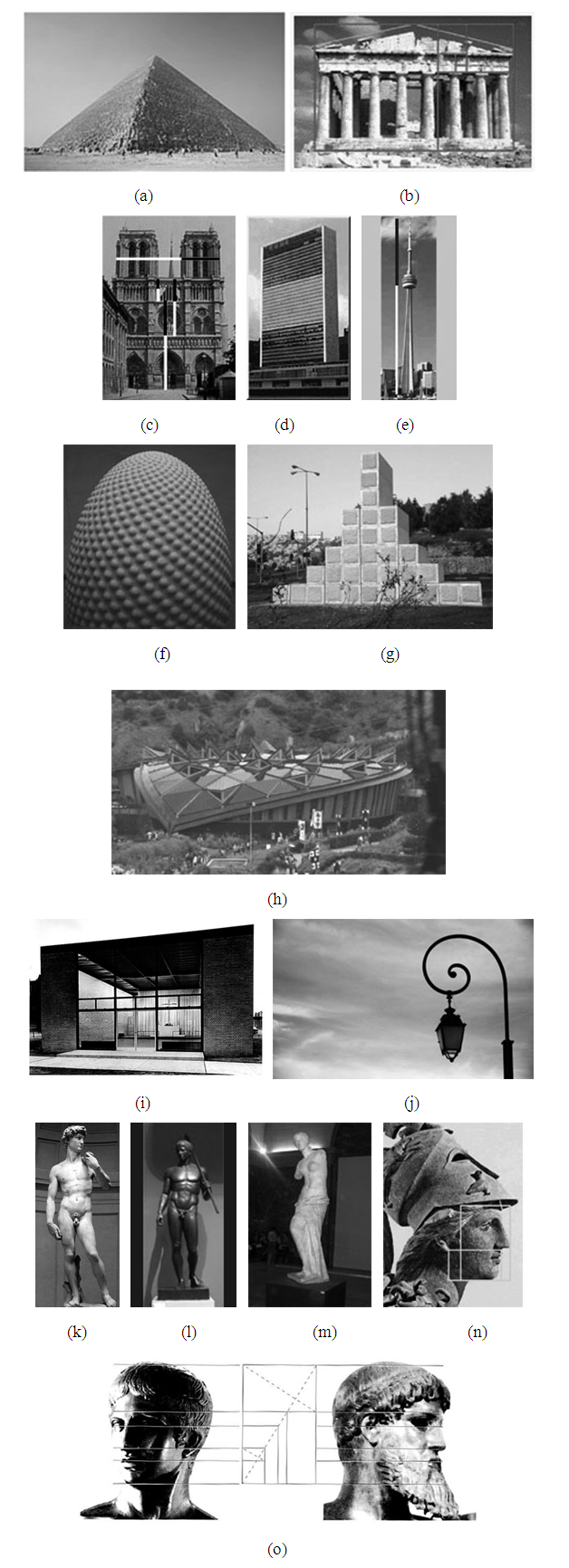Figure 25. Golden ratio in artifacts and manmade structures, (a) Pyramid structure, (b) Parthenon, (c) Notre Dame in Paris, (d) United Nation building, (e) Canadian National (CN) Tower, (f) Sculpture called The Seed designed by Peter Randall, (g) Australian sculptor Andrew Rogers's 50-ton stone and gold sculpture, entitled Golden Ratio, (h) Education Centre called The Core Eden Project, (i) Illinois Institute of Techonoloy Chapel, (j) Spiral design of a lamp post, (k) David Statue, (l) Doryphoros by Polykleitos, (m) Venus de Milo, (n) Statue of Athena, (o) Doryphoros and Artemision Face [36,47-51]
Peter Randall-Paige's artistic design was based on the spirals found in seeds and sunflowers and pinecones. Golden proportion was also used in the design of Notre Dame in Paris, which was built in the 1163 and 1250. In India, this proportion was also found in the construction of the Taj Mahal, which was completed in 1648. The United Nations building also shows the relation with the Golden ratio where the building is fitted with the three Golden Rectangles piled one over another. The CN Tower in Toronto, one of the tallest towers and freestanding structure of the world, has contains the golden ratio in its design. The ratio of observation deck at 342 meters to the total height of 553.33 is 1.6179, same as the Phi ratio. Figure 25 (c), (d) and (e) show the Notre Dame, the UN building and the CN tower.
In Doryphoros by Polykleitos, the ratio between the distances from navel to knee and from navel to sole is 1.61. The ratio of the distances from navel to sole and from navel to head is also 1.61. Australian sculptor Andrew Rogers's 50-ton stone and gold sculpture, entitled Golden Ratio, is donated and installed outdoors in Jerusalem, shown in Figure 25 (g). The height of each stack of stones, beginning from either end and moving toward the centre is the beginning of the Fibonacci sequence, 1, 1, 2, 3, 5 and 8. A geometrical analysis of the Great Mosque of Kairouan, built by Uqba ibn Nafi c. 670 A.D., reveals a consistent application of the golden ratio throughout the design. The analysis was done by Boussora and Mazouz who said that the golden ratio was found in the overall proportion of the plan and in the dimensioning of the prayer space, the court, and the minaret. The Stuppa of Borobudur in Java, Indonesia, the largest known Buddhist stupa was built in eighth to ninth century AD, has the dimension of the square base related to the diameter of the largest circular terrace as 1.618:1. According to the official tourism page of Buenos Aires, Argentina, the ground floor of the Palacio Barolo (1923), designed by Italian architect Mario Palanti, was built based on the golden section.
The Venus de Milo sculpture, shown in Figure 25 (m), was carved by the Greek sculptor Alexandros which is strictly to the Golden Proportion. On the face of the Statue of Athena, Figure 25 (n), the first Golden Ratio is the length from the front head to the ear opening compared with the length from the forehead to the chin. The second one appears in the ratio of the length from the nostril to the earlobe compare with the length from the nostril to the chin.
The Golden Section is a law of proportionality that occurs frequently in nature and its uses are useful in particle Arts. This was first developed by a Roman writer, architect and engineer, Marcus Vitruvius Pollio, which is most famously known from Leonardo Da Vinci's drawing 'The Divine Proportion'. Some studies of the Acropolis, including the Parthenon, conclude that many of its proportions approximate to the golden ratio. The Parthenon's facade as well as elements of its facade can be circumscribed by golden rectangles, which might indicate that their architects were aware of the golden ratio and consciously employed it in their designs. It is also possible that the architects used their own sense of good proportion, and that this led to some proportions that closely approximate the golden ratio. The Swiss architect Le Corbusier, famous for his contributions to the modern international style, centred his design philosophy on systems of harmony and proportion. His faith in the mathematical order of the universe was closely bound to the golden ratio and the Fibonacci series. He explicitly used the golden ratio in his system for the scale of architectural proportion. He saw this system as a continuation of the long tradition of Vitruvius, Leonardo da Vinci’s “Vitruvian Man”, the work of Leon Battista Alberti and others who used the proportions of the human body to improve the appearance and function of architecture. Le Corbusier sectioned his model human body’s height at the navel with the two sections in golden ratio, then subdivided those sections in golden ratio at the knees and throat. He used these golden ratio proportions in the Modulor system, a harmonious measure to the human scale, which was exemplified in his Villa Stein in Garches. The villa's rectangular ground plan, elevation, and inner structure closely approximate to the golden rectangles. Another Swiss architect, Mario Botta also applied the ratio in many of his designs on geometric figures. Several private houses he designed in Switzerland are composed of squares and circles, cubes and cylinders. In a house he designed in Origlio, has the golden ratio in the proportion between the central section and the side sections of the house.
Existence of the Golden Section in inanimate and artistic endeavours is more pleasing to human eye and creates a feeling of satisfaction and harmony within an image. As the Golden Section is found in the design and beauty of nature, it can also be used to achieve beauty and balance in the design of art. The Golden Section was used extensively by Leonardo Da Vinci. All the key dimensions of the room and the table in Da Vinci's "The Last Supper" were based on the Golden Section, which was known in the Renaissance period as The Divine Proportion. In "The Sacrament of the Last Supper," Salvador Dali framed his painting in a golden rectangle [47,48]. Following Da Vinci's lead, Dali positioned the table exactly at the golden section of the height of his painting. He positioned the two disciples at Christ's side at the golden sections of the width of the composition. In addition, the windows in the background are formed by a large dodecahedron. Dodecahedrons consist of 12 pentagons, which exhibit Phi relationships in their proportions. Figure 26 (b) and (e) show the two famous paintings, The Last Supper and The Sacrament of the Last Supper [1,4,47,48].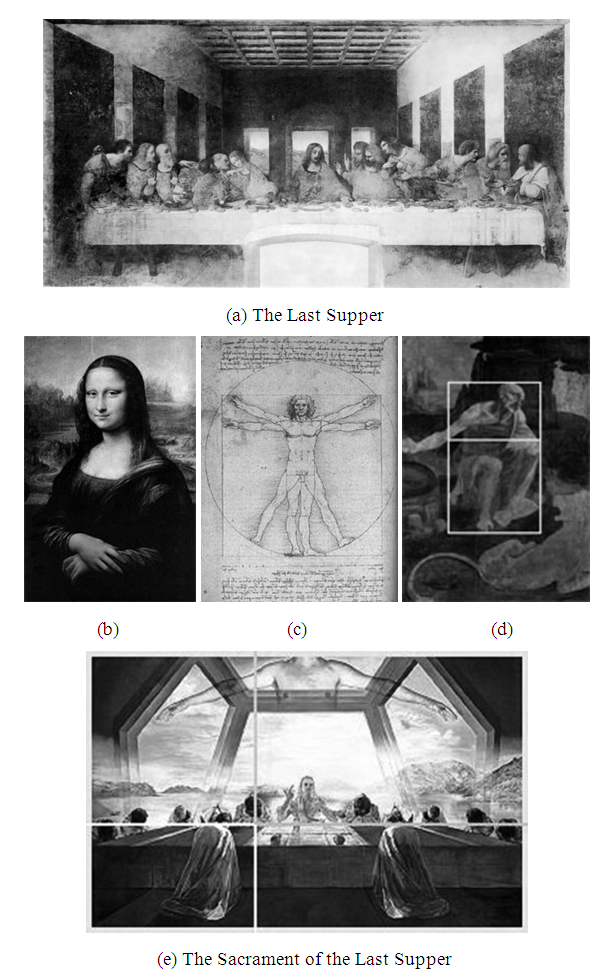Figure 26. (a) The Last Supper, (b) Monalisa Painting, (c) Bionic Man, (d) Leonardo's unfinished "St. Jerome", and (e) Salvador Dalí’s painting The Sacrament of the Last Supper [1,4,36,47,48]
Leonardo Da Vinci's illustrations on The Divine Proportion and his views on some bodily proportions led some scholars to speculate that he incorporated the golden ratio in his own paintings. Some suggest that his Monalisa employs the golden ratio in its geometric equivalents. Whether Leonardo proportioned his paintings according to the golden ratio has been the subject of intense debate. The secretive Leonardo seldom disclosed the bases of his art, and retrospective analysis of the proportions in his paintings can never be conclusive. Mondrian also used the golden section extensively in some of his geometrical paintings.
Phi relationships in a colour spectrum produce rich, appealing colour combinations. Michael Semprevivo has introduced a concept called the PhiBar, which applies Phi relationships to frequencies or wavelengths in the spectrum of visible colours in light. Colours in the spectrum that are related by distances based on Phi or the golden section produce very rich and visually appealing combinations.
Golden proportion also found on the design of the Volkswagen Beetle, which was designed in 1997 by Jay Mays, Freeman Thomas, Peter Schreyer . The body fits nearly into the top half of a golden ellipse and the side windows also repeat the shape of the golden ellipse with the door, shown in Figure 27 (a), (b), (c) and (d). The front and rear view of the car are almost in a square with all surfaces symmetrical. Golden proportion appears on the design of some other cars shown in Figure 27 (e) and (f).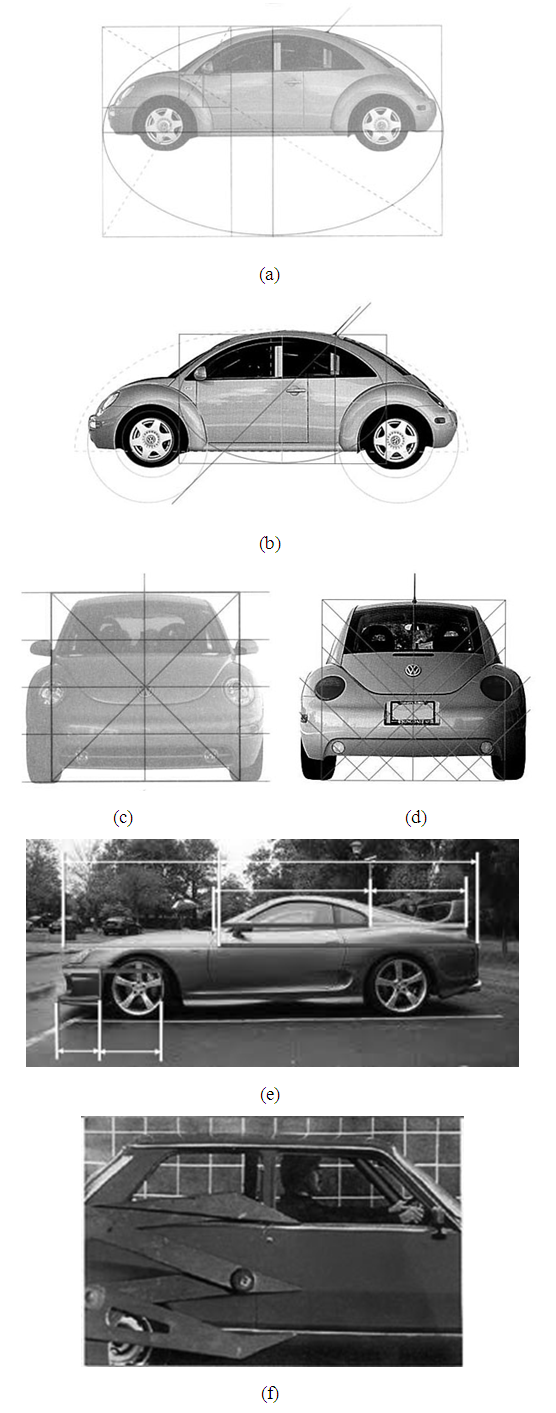Figure 27. Golden Ratio based design of cars, (a), (b), (c) and (d) Volkswagen Beetle, (e) Toyota Supra, and (f) Golden Section appears on a car’s door [1,47,48]
A Penrose tiling is a non-periodic tiling generated by an aperiodic set of prototiles named after Sir Roger Penrose . A Penrose tiling may be constructed to exhibit both reflection symmetry and five folded rotational symmetry. The first Penrose tiling, called P1, is an aperiodic set of six tiles, introduced by Roger Penrose in 1974 which was based on pentagons rather than squares. Any attempts to tile the plane with regular pentagons will necessarily leave gaps, but Johannes Kepler showed, in his Harmonices Mundi, that these gaps could be filled up by using pentagrams, decagons and related shapes. The number of prototiles of Penrose was reduced into two for the kite and dart tiling called P2 and the rhombus tiling named as P3. The rhombus tiling was independently discovered by Robert Ammann in 1976. The three types of Penrose tiling P1, P2 and P3 have many common features related to the pentagon and hence to the Golden Ratio.
Penrose's first tiling, P1, uses pentagons and three other shapes as a five-pointed "star" or a pentagram, a "boat" which is roughly 3/5 of a star and a "diamond". Penrose's second tiling uses quadrilaterals called the "kite" and "dart", which may be combined to make a rhombus. Both the kite and dart are composed of two triangles, called Robinson triangles. The kite is a quadrilateral whose four interior angles are 72, 72, 72, and 144 degrees. The kite may be bisected along its axis of symmetry to form a pair of acute Robinson triangles with angles of 36, 72 and 72 degrees, which are exactly same as the golden triangle. The dart is a non-convex quadrilateral with four interior angles as 36, 72, 36, and 216 degrees. The dart may be bisected along its axis of symmetry to form a pair of obtuse Robinson triangles having angles of 36, 36 and 108 degrees, which are also smaller to the golden triangles. The third tiling uses a pair of rhombuses with equal sides but different angles. The thin rhomb ‘t’ has four corners with angles of 36, 144, 36, and 144 degrees. The ‘t’ rhomb can be bisected along its short diagonal to form a pair of acute Robinson triangles. The thick rhomb ‘T’ has angles of 72, 108, 72, and 108 degrees and that also can be bisected along its long diagonal to form two acute Robinson triangles or golden triangles.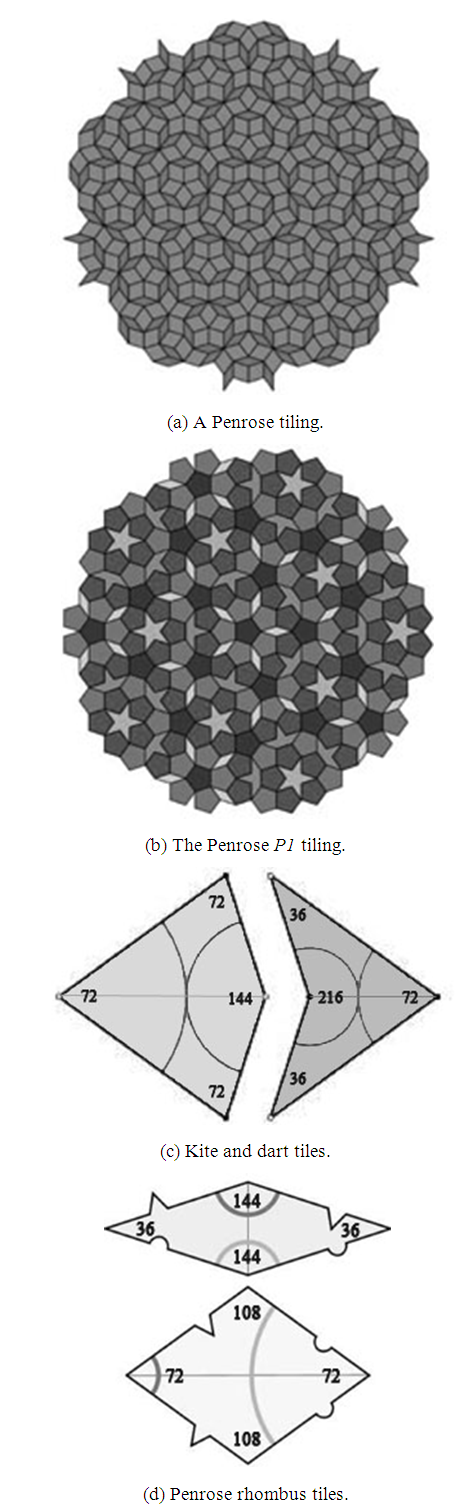Figure 28. Penrose tiling and tiles sizing, (a) An example of Penrose tiling, (b) The original pentagonal Penrose tiling (P1), (c) Tiles used in Kite and Dart tiling (P2) pattern, and (d) Tiles used in Rhombus tiling (P3) 

### 6. Conclusions

Using Fibonacci numbers, the Golden Ratio becomes a golden spiral, that plays an enigmatic role everywhere in the nature such as in shells, pine cones, the arrangement of seeds in a sunflower head and even galaxies. Adolf Zeising, a mathematician and philosopher, while studying the natural world, saw that the Golden Ratio is operating as a universal law. On the other hand, some scholars deny that the Greeks had any aesthetic association with golden ratio. Midhat J. Gazale says that until Euclid the golden ratio's mathematical properties were not studied. In the “Misconceptions about the Golden Ratio”, Dr. George Markowsky also discussed about some misconceptions of the properties and existence golden ratio  in various structures and design. Basically the Golden Ratio should not be considered as a convention to all circumstances like a law of nature but it needs deeper study and analysis to establish the relation with the ratio as it is a curiosity of researchers to fulfil the demand of this field of research. Some group of researchers in 1991 found an interesting rule in the decay profile of the RB type II Bursts that follows the golden ratio . Y.H. Chan and S.A.R. Abu-Baker used a new strategy called Modified Golden Ratio (MGR) and designed an algorithm for face boundary refinement and face extraction . Some other researchers also used the ratio to detect the face region in digital images . Micha Hofri and Zvi Rosberg was designed a protocol named as Golden Ratio Control Policy for Multiple Access Channel Network and achieved the cost closer to the lower bound for realistic parameters . Qing Xue and Phillip Sheu applied the golden section search method to find a set of collision free optimal paths for mobile robots . In recent years the research on humanoid robotic platform is spreading rapidly where some researchers suggest that the golden ratio based analysis and designed can be considered for the design of Android structure [34,40,41,44-46].
Basically, this study represents a qualitative review on Golden Proportion from ancient time to the present. The study also represents the mystery of various geometrical patterns and various dynamic rectangles. The proof of the equation of Phi based on the classical geometrical approach is also illustrated in this paper. Mainly the paper draws an extensive review on natural and artificial world to find out the relations with the Phi as the Golden and Devine Ratio.

### ACKNOWLEDGEMENTS

The authors would like to thank their honourable parents. They also would like to express their appreciations to the Ministry of Higher Education (MOHE), Malaysia, Research Management Centre (RMC), IIUM and Kulliyyah of Engineering, (KOE), IIUM, Malaysia.